Frequency Distribution - Presentation of Data, Business Mathematics & Statistics

# Frequency Distribution - Presentation of Data, Business Mathematics & Statistics - Business Mathematics and Statistics - B Com

FREQUENCY DISTRIBUTION

Frequency of a value of a variable is the number of times it occurs in a given series of observations. A tallysheet may be used to calculate the frequencies from the raw data (primary data not arranged in the Tabular form). A tally-mark (/) is put against the value when it occurs in the raw data. The following example shows how raw-data can be represented by a tally-sheet :

Example 2 : Raw data Marks in Mathematics of 50 students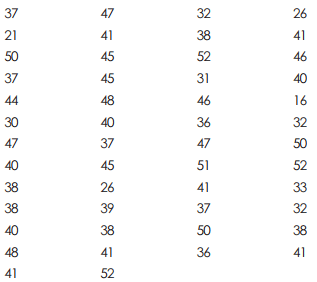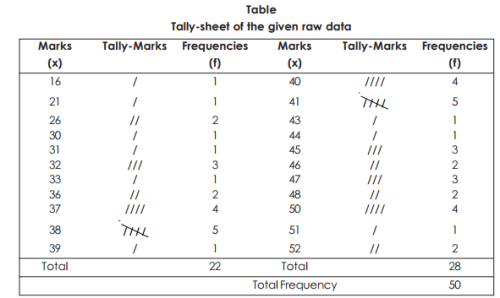Such a representation of the data is known as the Frequency Distribution. The number of classes should neither be too large nor too small. It should not exceed 20 but should not be less than 5, normally, depending on the number of observations in the raw data.

Group Frequency Distribution : When large masses of raw data are to be summarised and the identity of the individual observation or the order in which observations arise are not relevant for the analysis, we distribute the data into classes or categories and determine the number of individuals belonging to each class, called the class-frequency. A tabular arrangement of raw data by classes where the corresponding class-frequencies are indicated is known as Grouped Frequency distribution.

Grouped Frequency Distribution of Marks of 50 students in Mathematics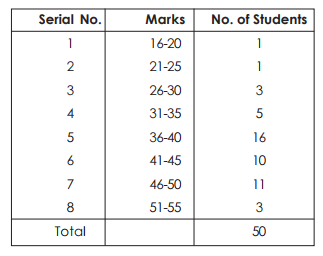Few Terms (associated with grouped frequency distribution) :
(a) Class-interval
(b) Class-frequency, total frequency
(c) Class-limits (upper and lower)
(d) Class boundaries (upper and lower)
(e) Mid-value of class interval (or class mark)
(f) Width of class interval
(g) Frequency denisty (
h) Percentage Frequency.

(a) Class-interval : In the above table, class intervals are 16-20, 21-25 …. etc. In all there are eight class-intervals. If, however, one end of class-interval is not given then it is known as open-end class. For example, less than 10, 10-20, 20-30, 30 and above. The class-interval having zero frequency is know as empty class.
(b) Class frequency : The number of observations (frequency) in a particular class-interval is known as class-frequency. In the table, for the class-interval 26-30, class frequency is 3 and so on. The sum of all frequencies is total frequency. Here in the table total frequency is 50.
(c) Class limits : The two ends of a class-interval are called class-limits.
(d) Class boundaries : The class boundaries may be obtained from the class limits as follows : Lower class-boundary = lower class limit – ½ d Upper class-boundary = upper class limit + ½ d Where d = common difference between upper class of any class-interval with the lower class of the next class-interval. In the table d = 1.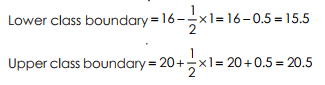Again, for the next class-interval, lower class-boundary = 20.5, upper class boundary = 25.5 and so on.

(e) Mid value : (or class mark). It is calculated by adding the two class limits divided by 2. In the above table : for the first class-interval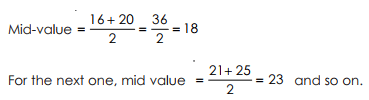(f) Width : The width (or size) of a class interval is the difference between the class-boundaries (not class limits)
Width = Upper class boundary – lower class boundary

For the first class, width = 20.5 – 15.5 = 5
For the second class width = 25.5 – 20.5 = 5, so on.

(g) Frequency density : It is the ratio of the class frequency to the width of that

class-interval i.e. frequency density =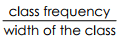For the first class frequency density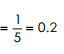For the third class frequency density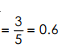(h) Percentage frequency : It is the ratio of class-frequency to total frequency expressed as percentage

i.e. percentage frequency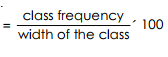In the table for the frequency 5, % frequency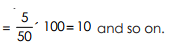Cumulative

Frequency distribution : As the name suggests, in this distribution, the frequencies are cumulated. This is prepared from a grouped frequency distribution showing the class boundaries by adding each frequency to the total of the previous one, or those following it. The former is termed as Cumulative frequency of less than type and the latter, the cumulative frequency of greater than type.

Example 3 : The following is an array of 65 marks obtained by students in a certain examination : –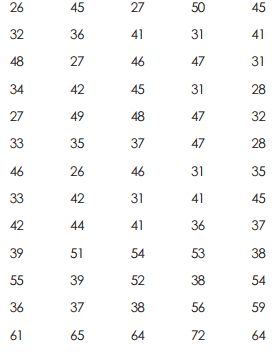Draw up a frequency distribution table classified on the basis of marks with class-intervals of 5.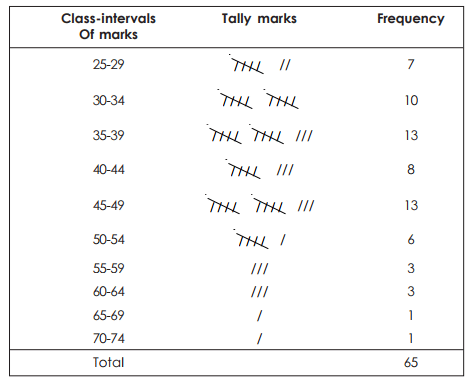Now the required frequency distribution is shown below :
Frequency distribution of marks obtained by 65 students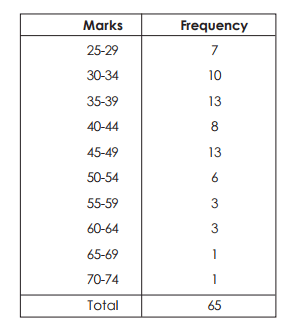The document Frequency Distribution - Presentation of Data, Business Mathematics & Statistics | Business Mathematics and Statistics - B Com is a part of the B Com Course Business Mathematics and Statistics.
All you need of B Com at this link: B Com

115 videos|142 docs

## FAQs on Frequency Distribution - Presentation of Data, Business Mathematics & Statistics - Business Mathematics and Statistics - B Com

 1. What is a frequency distribution?Ans. A frequency distribution is a way of organizing and presenting data in tabular or graphical form to show the number of times each value or range of values occurs in a dataset. It provides a summary of the data by showing the frequency or count of each value or range.
 2. How is a frequency distribution useful in business mathematics and statistics?Ans. Frequency distribution is useful in business mathematics and statistics as it helps in analyzing and understanding the patterns, trends, and distribution of data. It allows businesses to identify the most common or frequent values, outliers, and potential areas of improvement. It is also an essential tool for making data-driven decisions and conducting further statistical analysis.
 3. What are the steps involved in creating a frequency distribution?Ans. The steps involved in creating a frequency distribution are as follows: 1. Determine the range of values: Identify the minimum and maximum values in the dataset. 2. Determine the number of intervals or classes: Decide on the number of intervals or classes that will be used to group the data. 3. Determine the width of each interval: Calculate the width of each interval by dividing the range by the number of intervals. 4. Create the intervals: Construct the intervals by starting from the minimum value and incrementing by the width. 5. Count the frequencies: Count the number of values that fall within each interval. 6. Present the data: Present the frequencies in a tabular or graphical form, such as a histogram or bar graph.
 4. What are the advantages of using a frequency distribution?Ans. The advantages of using a frequency distribution are as follows: 1. Data organization: It provides a structured way of organizing data, making it easier to understand and analyze. 2. Data summary: It summarizes the data by showing the frequency or count of each value or range, giving a quick overview of the dataset. 3. Pattern identification: It helps in identifying patterns, trends, and outliers in the data, which can be useful in decision-making and problem-solving. 4. Data visualization: It allows for the visual representation of data through graphs and charts, making it easier to interpret and communicate. 5. Statistical analysis: It serves as a basis for further statistical analysis, such as calculating measures of central tendency and dispersion.
 5. How can a frequency distribution be used to make business decisions?Ans. A frequency distribution can be used to make business decisions by providing insights into the distribution of data. For example: 1. Identifying popular products or services: By analyzing the frequency distribution of sales data, businesses can identify the most popular products or services and allocate resources accordingly. 2. Analyzing customer preferences: By examining the frequency distribution of customer feedback or survey responses, businesses can understand customer preferences and tailor their products or services accordingly. 3. Detecting outliers or anomalies: By studying the frequency distribution of financial data, such as expenses or revenues, businesses can detect outliers or anomalies that may require further investigation or action. 4. Evaluating process performance: By analyzing the frequency distribution of process data, such as manufacturing defects or customer complaints, businesses can identify areas of improvement and make necessary adjustments to enhance efficiency and quality. 5. Forecasting demand: By analyzing the frequency distribution of historical sales data, businesses can forecast future demand and make informed decisions regarding production, inventory, and pricing strategies.

115 videos|142 docsExplore Courses for B Com examSignup to see your scores go up within 7 days! Learn & Practice with 1000+ FREE Notes, Videos & Tests.
10M+ students study on EduRev
Track your progress, build streaks, highlight & save important lessons and more!
Related Searches

,

,

,

,

,

,

,

,

,

,

,

,

,

,

,

,

,

,

,

,

,

,

,

,

;